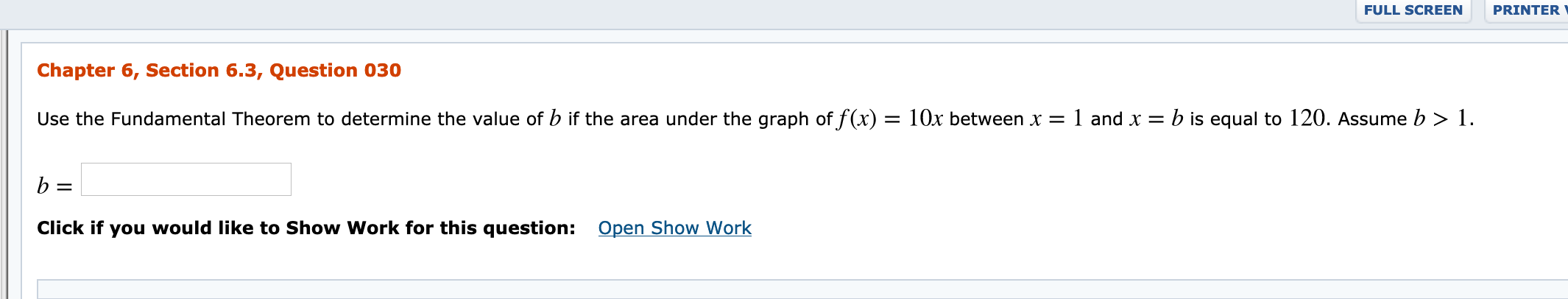# FULL SCREENPRINTERChapter 6, Section 6.3, Question 030Use the Fundamental Theorem to determine the value of b if the area under the graph of f(x) = 10x between x = 1 and x = b is equal to 120. Assume b > 1.Open Show WorkClick if you would like to Show Work for this question:

Question
7 viewshelp_outlineImage TranscriptioncloseFULL SCREEN PRINTER Chapter 6, Section 6.3, Question 030 Use the Fundamental Theorem to determine the value of b if the area under the graph of f(x) = 10x between x = 1 and x = b is equal to 120. Assume b > 1. Open Show Work Click if you would like to Show Work for this question: fullscreen
check_circle

Step 1

Given:

f (x) = 10x between x = 1 and x = b is equal to 120.

Step 2

Concept used:

Fundamental Theorem of calculus states that if f is continuous on the c...

### Want to see the full answer?

See Solution

#### Want to see this answer and more?

Solutions are written by subject experts who are available 24/7. Questions are typically answered within 1 hour.*

See Solution
*Response times may vary by subject and question.
Tagged in

### Integration